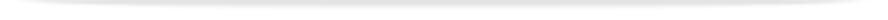units

ENG1091

Faculty of Engineering

# Undergraduate - UnitENG1091 - Mathematics for engineering

This unit entry is for students who completed this unit in 2013 only. For students planning to study the unit, please refer to the unit indexes in the the current edition of the Handbook. If you have any queries contact the managing faculty for your course or area of study.print version

## 6 points, SCA Band 2, 0.125 EFTSL

To find units available for enrolment in the current year, you must make sure you use the indexes and browse unit tool in the current edition of the Handbook.

 Level Undergraduate Faculty Faculty of Engineering Offered Clayton First semester 2013 (Day)Sunway First semester 2013 (Day)Clayton Second semester 2013 (Day)Sunway Second semester 2013 (Day)Sunway October intake 2013 (Day) Coordinator(s) Sem 1: Dr Leo Brewin (Clayton); Associate Professor Lan Boon Leong (Sunway)

### Synopsis

Vector algebra and geometry: equations of lines and planes. Linear algebra: matrix operations, systems of linear equations, eigenvalues and eigenvectors. Calculus: logarithmic differentiation, improper integrals, integration by parts. Sequences and series: convergence, power series, Taylor polynomials. Ordinary differential equations: first order, second order with constant coefficients, boundary value problems, systems of ODEs. Multivariable calculus: partial derivatives, directional derivatives, chain rule, maxima and minima.

### Outcomes

On completing this unit, students will be able to calculate cross products of vectors, and use vectors to represent lines and planes; perform matrix algebra; solve systems of linear equations and find eigenvalues and eigenvectors in simple cases; use hyperbolic functions; perform logarithmic differentiation; establish the convergence of improper integrals, and use further techniques of integration, including integration by parts; establish the convergence of numeric and power series, construct Taylor series and use Taylor polynomials to approximate functions; solve first order ordinary differential equations, including the techniques of exact integration, separable variables and integrating factor; and systems of ordinary differential equations; solve 2nd order linear differential equations with constant coefficients; set up differential equations with initial or boundary conditions to model simple engineering problems; calculate partial derivatives, use the grad vector to find directional derivatives, use chain rule, calculate small error using the total differential, and find maximum and minimum values of two-variable functions.

### Assessment

Assignments and test: 30%
Examination (3 hours): 70%

### Chief examiner(s)

Dr Leo Brewin (Sem 1 + 2)
Dr Leo Brewin (Sunway October intake 2013)

### Contact hours

3 hours lectures, 2 hours practice classes and 7 hours of private study per week

### Prerequisites

VCE Specialist Mathematics (or ENG1090 or equivalent)

### Prohibitions

ENG1902, MTH1030, MAT1085